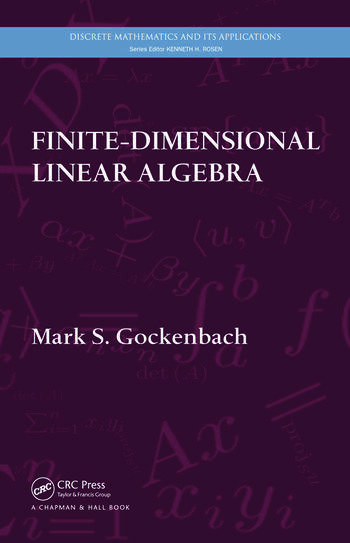# Finite-Dimensional Linear Algebra

## 1st Edition

Mark S. Gockenbach

CRC Press
Published May 6, 2010
Textbook - 672 Pages - 50 B/W Illustrations
ISBN 9781439815632 - CAT# K10803
Series: Discrete Mathematics and Its Applications

For Instructors Request Inspection Copy

USD\$130.00

FREE Standard Shipping!

## Preview

### Summary

Linear algebra forms the basis for much of modern mathematics—theoretical, applied, and computational. Finite-Dimensional Linear Algebra provides a solid foundation for the study of advanced mathematics and discusses applications of linear algebra to such diverse areas as combinatorics, differential equations, optimization, and approximation.

The author begins with an overview of the essential themes of the book: linear equations, best approximation, and diagonalization. He then takes students through an axiomatic development of vector spaces, linear operators, eigenvalues, norms, and inner products. In addition to discussing the special properties of symmetric matrices, he covers the Jordan canonical form, an important theoretical tool, and the singular value decomposition, a powerful tool for computation. The final chapters present introductions to numerical linear algebra and analysis in vector spaces, including a brief introduction to functional analysis (infinite-dimensional linear algebra).

Drawing on material from the author’s own course, this textbook gives students a strong theoretical understanding of linear algebra. It offers many illustrations of how linear algebra is used throughout mathematics.

#### Instructors

We provide complimentary e-inspection copies of primary textbooks to instructors considering our books for course adoption.